# High School Math : Acute / Obtuse Triangles

## Example Questions

← Previous 1 3 4 5 6

### Example Question #1 : Acute / Obtuse Triangles

Two similiar triangles have a ratio of perimeters of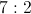.

If the smaller triangle has sides of 3, 7, and 5, what is the perimeter of the larger triangle.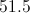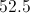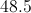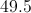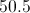Explanation:

Adding the sides gives a perimeter of 15 for the smaller triangle. Multipying by the given ratio of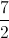, yields 52.5.

### Example Question #1 : Acute / Obtuse Isosceles Triangles

Two sides of an isosceles triangle are 20 and 30. What is the difference of the largest and the smallest possible perimeters?

0

30

10

15

10

Explanation:

The trick here is that we don't know which is the repeated side. Our possible triangles are therefore 20 + 20 + 30 = 70 or 30 + 30 + 20 = 80.  The difference is therefore 80 – 70 or 10.

### Example Question #3 : Acute / Obtuse Triangles

Two similiar triangles exist where the ratio of perimeters is 4:5 for the smaller to the larger triangle. If the larger triangle has sides of 6, 7, and 12 inches, what is the perimeter, in inches, of the smaller triangle?

25

23

18

20

20

Explanation:

The larger triangle has a perimeter of 25 inches. Therefore, using a 4:5 ratio, the smaller triangle's perimeter will be 20 inches.

### Example Question #4 : Acute / Obtuse Triangles

If a = 7 and b = 4, which of the following could be the perimeter of the triangle?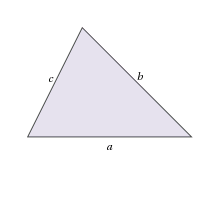I. 11

II. 15

III. 25

II and III Only

I, II and III

II Only

I and II Only

I Only

II Only

Explanation:

Consider the perimeter of a triangle:

P = a + b + c

Since we know a and b, we can find c.

In I:

11 = 7 + 4 + c

11 = 11 + c

c = 0

Note that if c = 0, the shape is no longer a trial. Thus, we can eliminate I.

In II:

15 = 7 + 4 + c

15 = 11 + c

c = 4.

This is plausible given that the other sides are 7 and 4.

In III:

25 = 7 + 4 + c

25 = 11 + c

c = 14.

It is not possible for one side of a triangle to be greater than the sum of both of the other sides, so eliminate III.

Thus we are left with only II.

### Example Question #5 : Acute / Obtuse Triangles

Solve for. (Not drawn to scale).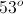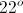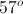Explanation:

The angles of a triangle must add to 180o. In the triangle to the right, we know one angle and can find another using supplementary angles.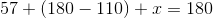Now we only need to solve for.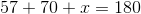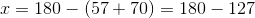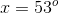### Example Question #6 : Acute / Obtuse Triangles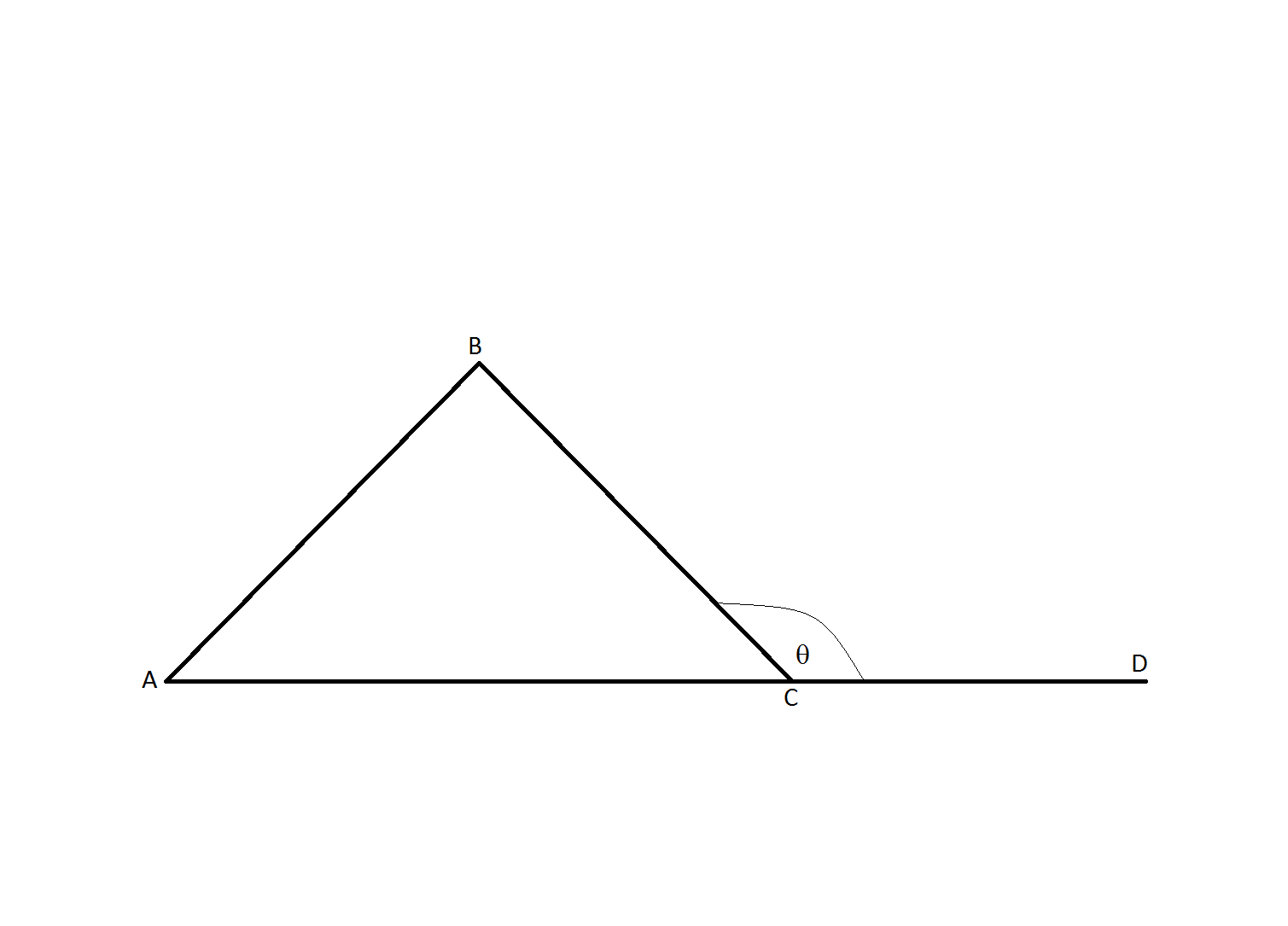If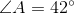and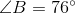, what is the measure of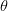?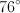Not enough information to solve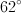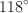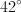Explanation:

All of the interior angles of a triangle add up to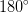.

Ifand, then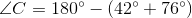Therefore,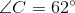Now,will equal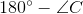because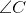andform a straight line.  Therefore,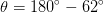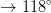Also, by definition, the angle of an exterior angle of a triangle is equal to the measure of the two interior angles opposite of it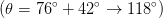.

### Example Question #7 : Acute / Obtuse Triangles

Two interior angles in an obtuse triangle measure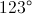and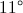. What is the measurement of the third angle.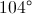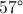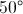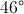Explanation:

Interior angles of a triangle always add up to 180 degrees.

### Example Question #4 : How To Find The Measure Of An Angle

In a given triangle, the angles are in a ratio of 1:3:5.  What size is the middle angle?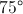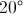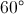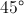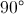Explanation:

Since the sum of the angles of a triangle is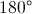, and given that the angles are in a ratio of 1:3:5, let the measure of the smallest angle be, then the following expression could be written: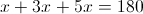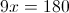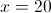If the smallest angle is 20 degrees, then given that the middle angle is in ratio of 1:3, the middle angle would be 3 times as large, or 60 degrees.

### Example Question #1 : How To Find An Angle In An Acute / Obtuse Isosceles Triangle

Triangle ABC has angle measures as follows: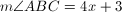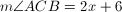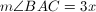What is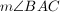?

90

19

57

79

44

57

Explanation:

The sum of the measures of the angles of a triangle is 180.

Thus we set up the equation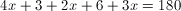After combining like terms and cancelling, we have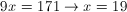Thus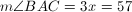### Example Question #2 : How To Find An Angle In An Acute / Obtuse Isosceles Triangle

The base angle of an isosceles triangle is five more than twice the vertex angle.  What is the base angle?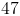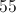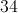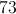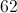Explanation:

Every triangle has 180 degrees.  An isosceles triangle has one vertex angle and two congruent base angles.

Let= the vertex angle and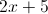= the base angle

So the equation to solve becomes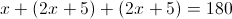Thus the vertex angle is 34 and the base angles are 73.

← Previous 1 3 4 5 6